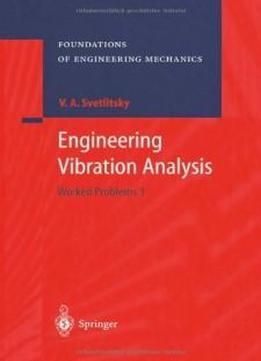# Engineering Vibration Analysis: Worked Problems 1 (foundations Of Engineering Mechanics) by Valery A. Svetlitsky / 2010 / English / PDF

Theory of vibrations belongs to principal subjects needed for training mechani­ cal engineers in technological universities. Therefore, the basic goal of the mono­ graph "Advanced Theory of Vibrations 1" is to help students studying vibration theory for gaining experience in application of this theory for solving particular problems. Thus, while choosing the problems and methods to solve them, the close attention was paid to the applied content of vibration theory. The monograph is devoted to systems with a single degree of freedom and sys­ tems with a finite number of degrees of freedom. In particular, problems are for­ mulated associated with determination of frequencies and forms of vibrations, study of forced vibrations, analysis of both stable and unstable vibrations (includ­ ing those caused by periodic but anharmonic forces). The problems of nonlinear vibrations and of vibration stability, and those related to seeking probabilistic characteristics for solutions to these problems in the case of random forces are also considered. Problems related to parametric vibrations and statistical dynamics of mechanical systems, as well as to determination of critical parameters and of dy­ namic stability are also analyzed. As a rule, problems presented in the monograph are associated with particular mechanical systems and can be applied for current studies in vibration theory. Al­ lowing for interests of students independently studying theory of vibrations, the majority of problems are supplied with either detailed solutions or algorithms of the solutions.

Theory of vibrations belongs to principal subjects needed for training mechani­ cal engineers in technological universities. Therefore, the basic goal of the mono­ graph "Advanced Theory of Vibrations 1" is to help students studying vibration theory for gaining experience in application of this theory for solving particular problems. Thus, while choosing the problems and methods to solve them, the close attention was paid to the applied content of vibration theory. The monograph is devoted to systems with a single degree of freedom and sys­ tems with a finite number of degrees of freedom. In particular, problems are for­ mulated associated with determination of frequencies and forms of vibrations, study of forced vibrations, analysis of both stable and unstable vibrations (includ­ ing those caused by periodic but anharmonic forces). The problems of nonlinear vibrations and of vibration stability, and those related to seeking probabilistic characteristics for solutions to these problems in the case of random forces are also considered. Problems related to parametric vibrations and statistical dynamics of mechanical systems, as well as to determination of critical parameters and of dy­ namic stability are also analyzed. As a rule, problems presented in the monograph are associated with particular mechanical systems and can be applied for current studies in vibration theory. Al­ lowing for interests of students independently studying theory of vibrations, the majority of problems are supplied with either detailed solutions or algorithms of the solutions.

views: 628